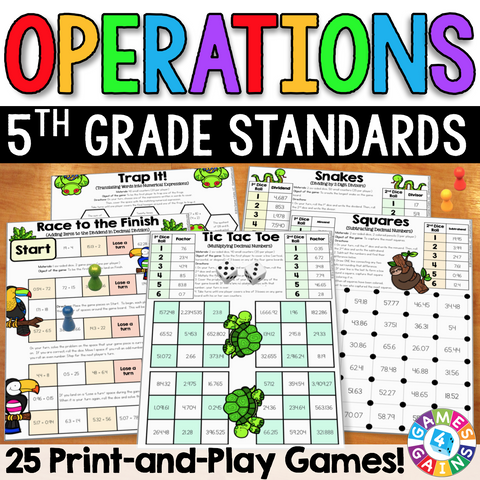• \$745

These games make 5th grade operations standards so much fun to practice! This 5th Grade Operations Games Pack contains 25 fun and engaging printable board games to help students to practice 5th grade whole number multiplication, whole number division, decimal addition, decimal subtraction, decimal multiplication, decimal division, and order of operations standards.

This pack includes 25 DIFFERENTIATED games that cover the following 5th grade operations skills:

Whole Number Multiplication:
• Estimating Products
• Multiplying 3- and 4-Digit by 2-Digit Numbers
• Multiplying Larger Numbers by 3-Digit Numbers

Whole Number Division:
• Estimating Quotients
• Dividing by 2-Digit Divisors
• Interpreting Remainders

• Estimating Decimal Sums
• Finding the Unknown in Decimal Addition and Subtraction

Decimal Subtraction:
• Estimating Decimal Differences
• Subtracting Decimals
• Subtracting Decimal Numbers from Whole Numbers

Decimal Multiplication:
• Estimating Decimal Products
• Multiplying Decimals by Whole Numbers
• Multiplying Decimals by Decimals
• Multiplying Decimals with Zeros in the Product

Decimal Division:
• Estimating Decimal Quotients
• Dividing Decimals by Whole Numbers
• Dividing Decimals by Decimals
• Adding Zeros to the Dividend in Decimal Division
• Finding the Unknown in Decimal Multiplication and Division

Order of Operations:
• Translating Simple Expressions
• Evaluating Expressions with 2 Operations
• Evaluating Expressions with 3 or More Operations

These games are so simple to use and require very minimal prep - just print, grab some basic materials, and play! They are perfect to use in math centers or as extension activities when students complete their work!

Included with this 5th Grade Operations Games Pack:
• Instructions for play
• 25 printable games
• Answer keys for all of the games

These 5th Grade Operations Games support the following Common Core math standards:

CCSS.MATH.CONTENT.5.NBT.B.5
Fluently multiply multi-digit whole numbers using the standard algorithm.

CCSS.MATH.CONTENT.5.NBT.B.6
Find whole-number quotients of whole numbers with up to four-digit dividends and two-digit divisors.

CCSS.MATH.CONTENT.5.NBT.B.7
Add, subtract, multiply, and divide decimals to hundredths.

CCSS.MATH.CONTENT.5.OA.A.1
Use parentheses, brackets, or braces in numerical expressions, and evaluate expressions with these symbols.

CCSS.MATH.CONTENT.5.OA.A.2
Write simple expressions that record calculations with numbers, and interpret numerical expressions without evaluating them.